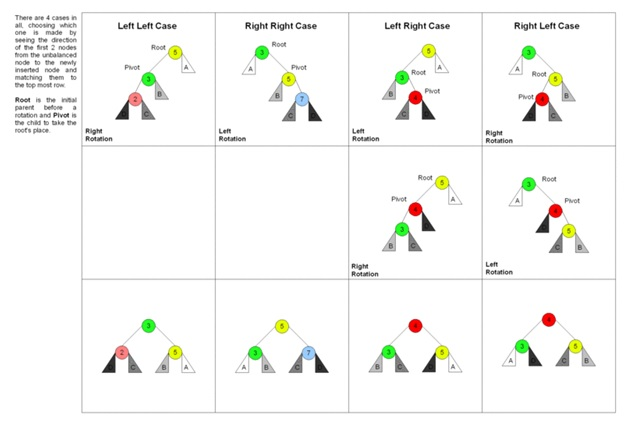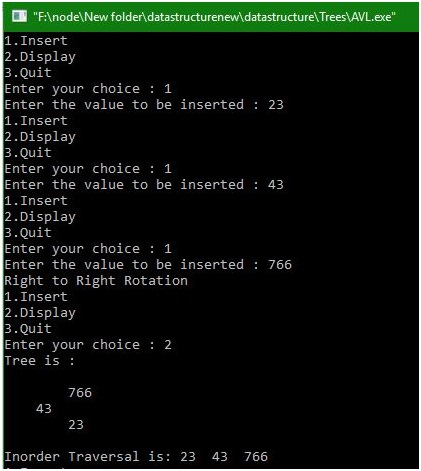# Insertion in AVL Tree using C program

Here, we are implementing a C program that will insert value (item) to an AVL Tree.
Submitted by Manu Jemini, on January 01, 2018

An AVL tree is a type of binary search tree, named after their inventors Adelson-Velskii and Landis. In AVL tree every node has to hold basic rules Binary Search tree i.e. the Value of parent node should be greater than the value of child node and smaller than equal to the value of right child node.

The AVL tree has another rule, which makes it better than BST, i.e. the height of any node in the tree should not exceed the limit -1, 1 inclusively. The Height of any node is determined by the difference of the level of height of left sub-tree and right sub-tree. AVL keeps track of height of every node and it updates after every insertion.

The Insertion of a new node in AVL happens the same way as of BST. Saying so, the value of the new node will compare with the root node and according to the result of the comparison, it will keen on comparing, till it finds the right spot.

After Insertion, there is a possibility that the height of one or more node can get out of the range. At that time we implement rotation in AVL tree.Image source: https://i.stack.imgur.com/GJFMj.gif

There are 4 cases given in above example which are Left-Left, Right-Right, Left-Right, and Right –Left.

## C program to implement ‘insertion in AVL Tree’

```#include<stdio.h>
#include<malloc.h>

typedef enum { FALSE ,TRUE } ;
struct node
{
int info;
int balance;
struct  node *lchild;
struct  node *rchild;
};

struct node *insert (int , struct node *, int *);
struct node* search(struct node *,int);

struct node* search(struct node *ptr,int info)
{
if(ptr!=NULL)
if(info < ptr->info)
ptr=search(ptr->lchild,info);
else if( info > ptr->info)
ptr=search(ptr->rchild,info);
return(ptr);
}/*End of search()*/

struct node *insert (int info, struct node *pptr, bool *ht_inc)
{
struct node *aptr;
struct node *bptr;

if(pptr==NULL)
{
pptr = (struct node *) malloc(sizeof(struct node));
pptr->info = info;
pptr->lchild = NULL;
pptr->rchild = NULL;
pptr->balance = 0;
*ht_inc = TRUE;
return (pptr);
}

if(info < pptr->info)
{
pptr->lchild = insert(info, pptr->lchild, ht_inc);
if(*ht_inc==TRUE)
{
switch(pptr->balance)
{
case -1: /* Right heavy */
pptr->balance = 0;
*ht_inc = FALSE;
break;
case 0: /* Balanced */
pptr->balance = 1;
break;
case 1: /* Left heavy */
aptr = pptr->lchild;
if(aptr->balance == 1)
{
printf("Left to Left Rotation\n");
pptr->lchild= aptr->rchild;
aptr->rchild = pptr;
pptr->balance = 0;
aptr->balance=0;
pptr = aptr;
}
else
{
printf("Left to right rotation\n");
bptr = aptr->rchild;
aptr->rchild = bptr->lchild;
bptr->lchild = aptr;
pptr->lchild = bptr->rchild;
bptr->rchild = pptr;

if(bptr->balance == 1 )
pptr->balance = -1;
else
pptr->balance = 0;
if(bptr->balance == -1)
aptr->balance = 1;
else
aptr->balance = 0;
bptr->balance=0;
pptr=bptr;
}
*ht_inc = FALSE;
}/*End of switch */
}/*End of if */
}/*End of if*/

if(info > pptr->info)
{
pptr->rchild = insert(info, pptr->rchild, ht_inc);
if(*ht_inc==TRUE)
{
switch(pptr->balance)
{
case 1: /* Left heavy */
pptr->balance = 0;
*ht_inc = FALSE;
break;
case 0: /* Balanced */
pptr->balance = -1;
break;
case -1: /* Right heavy */
aptr = pptr->rchild;
if(aptr->balance == -1)
{
printf("Right to Right Rotation\n");
pptr->rchild= aptr->lchild;
aptr->lchild = pptr;
pptr->balance = 0;
aptr->balance=0;
pptr = aptr;
}
else
{
printf("Right to Left Rotation\n");
bptr = aptr->lchild;
aptr->lchild = bptr->rchild;
bptr->rchild = aptr;
pptr->rchild = bptr->lchild;
bptr->lchild = pptr;
if(bptr->balance == -1)
pptr->balance = 1;
else
pptr->balance = 0;
if(bptr->balance == 1)
aptr->balance = -1;
else
aptr->balance = 0;
bptr->balance=0;
pptr = bptr;
}/*End of else*/
*ht_inc = FALSE;
}/*End of switch */
}/*End of if*/
}/*End of if*/

return(pptr);
}/*End of insert()*/

void display(struct node *ptr,int level)
{
int i;
if ( ptr!=NULL )
{
display(ptr->rchild, level+1);
printf("\n");
for (i = 0; i < level; i++)
printf("    ");
printf("%d", ptr->info);
display(ptr->lchild, level+1);
}/*End of if*/
}/*End of display()*/

void inorder(struct node *ptr)
{
if(ptr!=NULL)
{
inorder(ptr->lchild);
printf("%d  ",ptr->info);
inorder(ptr->rchild);
}
}/*End of inorder()*/

main()
{
bool ht_inc;
int info ;
int choice;
struct node *root = (struct node *)malloc(sizeof(struct node));
root =  NULL;

while(1)
{
printf("1.Insert\n");
printf("2.Display\n");
printf("3.Quit\n");
scanf("%d",&choice);
switch(choice)
{
case 1:
printf("Enter the value to be inserted : ");
scanf("%d", &info);
if( search(root,info) == NULL )
root = insert(info, root, &ht_inc);
else
printf("Duplicate value ignored\n");
break;
case 2:
if(root==NULL)
{
printf("Tree is empty\n");
continue;
}
printf("Tree is :\n");
display(root, 1);
printf("\n\n");
printf("Inorder Traversal is: ");
inorder(root);
printf("\n");
break;
case 3:
exit(1);
default:
printf("Wrong choice\n");
}/*End of switch*/
}/*End of while*/
}/*End of main()*/
```

Output